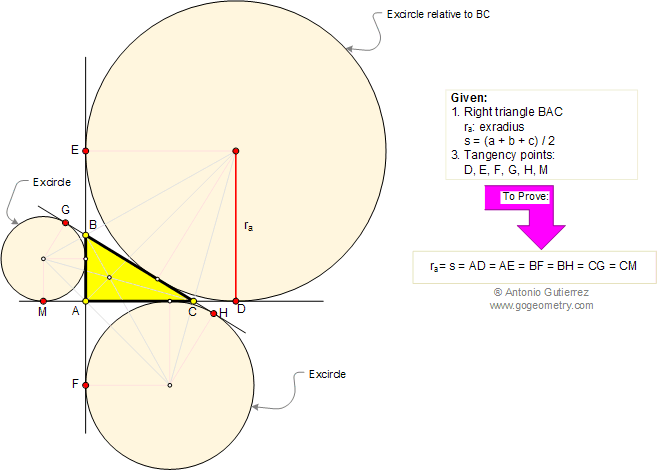Problem 201. Right Triangle, Excircles, Points of Tangency, Exradius, Semiperimeter
 In the figure below, BAC is a right triangle with the excircle of radius ra relative to the hypotenuse BC and the excircles relatives to the catheti (legs). If s is the semiperimeter and D, E, F, G, H, and M are the points of tangency, prove that ra = s = AD = AE = BF = BH = CG = CM. View or post a solution.Home | Geometry | Search | Problems | Art | 201-210 | Semiperimeter | Email | View or post a solution